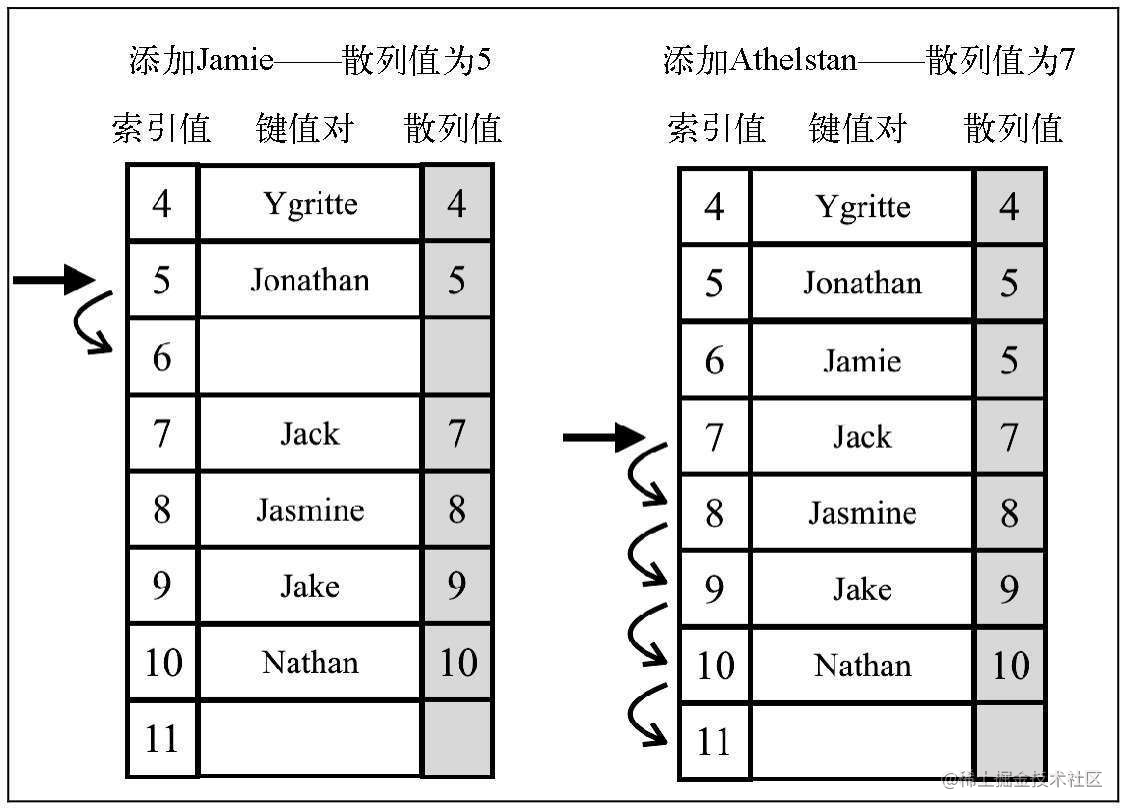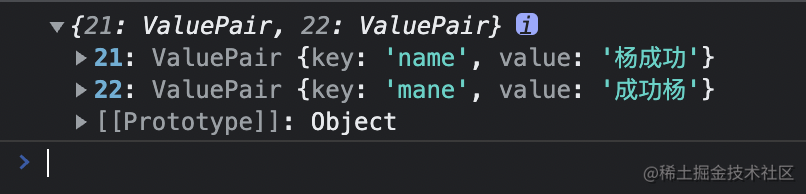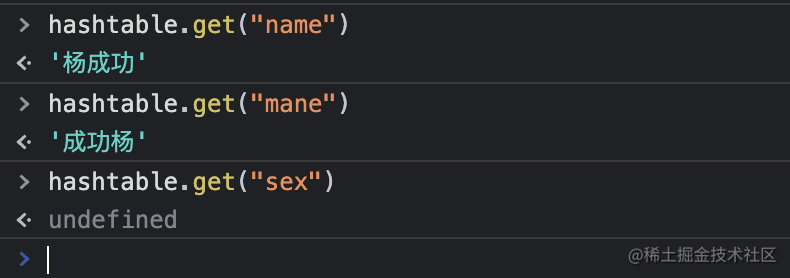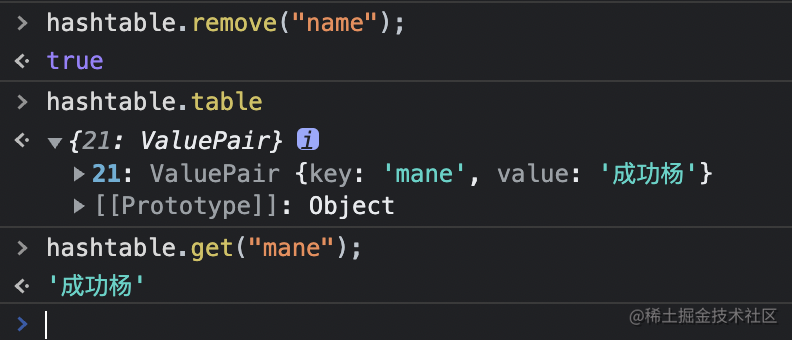# 线性探查法

## 线性探查法• 软删除
• 移动元素

`class HashTableLinearProbing extends HashMap {  constructor() {    super();    this.table = {};  }}`

### put 方法

put 方法用来添加元素，重写如下：

`put(key, value) {  if(key && value) {    let pos = this.hashCode(key);    while(this.table[pos]) {      pos++;    }    this.table[pos] = new ValuePair(key, value);    return true;  }  return undefined;}`

### get 方法

`get(key) {  let pos = this.hashCode(key);  if(this.table[pos]) {    if(this.table[pos].key === key) {      return this.table[pos].value    }    let index = pos + 1;    while(this.table[index] && this.table[index].key !== key) {      index++;    }    if(this.table[index] && this.table[index].key === key) {      return this.table[index].value    }  }  return undefined;}`

### remove 方法

remove 方法与 get 方法基本相同，核心都是找到某个元素。只不过 get 方法找到之后会返回，remove 方法则会删除。

`remove(key) {  let pos = this.hashCode(key);  if(this.table[pos]) {    if(this.table[pos].key === key) {      delete this.table[pos]      this.verifyRemoveSideEffect(key, pos)      return true    }    let index = pos + 1;    while(this.table[index] && this.table[index].key !== key) {      index++;    }    if(this.table[index] && this.table[index].key === key) {      delete this.table[index]      this.verifyRemoveSideEffect(key, index)      return true    }  }  return false;}`

`verifyRemoveSideEffect(key, pos) {  const hash = this.hashCode(key);  let index = pos + 1; // {2}  while (this.table[index] != null) { // {3}    const posHash = this.hashCode(this.table[index].key); // {4}    if (posHash <= hash || posHash <= pos) { // {5}      this.table[pos] = this.table[index]; // {6}      delete this.table[index];      pos = index;    }    index++;  }}`

`verifyRemoveSideEffect` 方法接收两个参数：被删除元素的 key 和被删除的位置 pos。

## 使用线性探查

`var hashtable = new HashTableLinearProbing();hashtable.put('name', '杨成功');hashtable.put('mane', '成功杨');console.log(hashtable.table);``hashtable.get('name');hashtable.get('mane');hashtable.get('sex');``hashtable.remove('name');console.log(hashtable.table);hashtable.get('mane');`